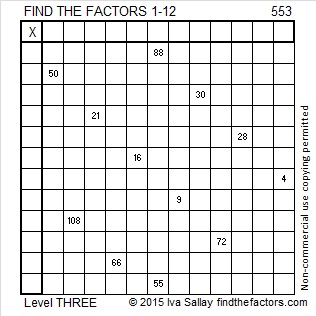# 553 and Level 3

79 is a factor of 553. If you start at 79 and go backwards adding all the prime numbers from 79 to 43, the sum will be 553. Do you know those prime numbers forwards and backwards well enough to do that?Print the puzzles or type the solution on this excel file: 12 Factors 2015-07-13

—————————————————————————————————

• 553 is a composite number.
• Prime factorization: 553 = 7 x 79
• The exponents in the prime factorization are 1 and 1. Adding one to each and multiplying we get (1 + 1)(1 + 1) = 2 x 2 = 4. Therefore 553 has exactly 4 factors.
• Factors of 553: 1, 7, 79, 553
• Factor pairs: 553 = 1 x 553 or 7 x 79
• 553 has no square factors that allow its square root to be simplified. √553 ≈ 23.51595—————————————————————————————————

A Logical Approach to solve a FIND THE FACTORS puzzle: Find the column or row with two clues and find their common factor. Write the corresponding factors in the factor column (1st column) and factor row (top row).  Because this is a level three puzzle, you have now written a factor at the top of the factor column. Continue to work from the top of the factor column to the bottom, finding factors and filling in the factor column and the factor row one cell at a time as you go.This site uses Akismet to reduce spam. Learn how your comment data is processed.